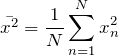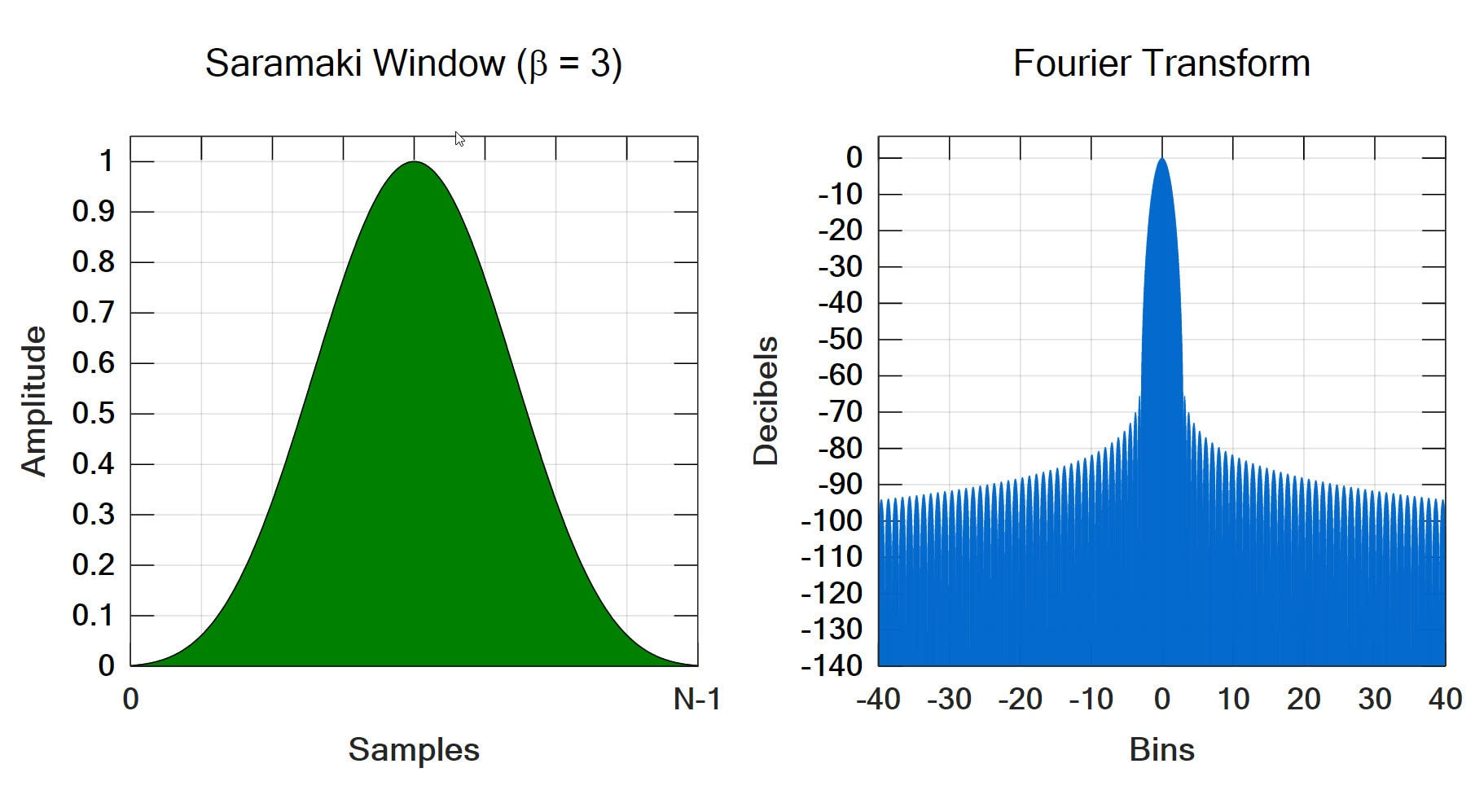# Mean Square

March 29, 2018

The mean-square value of a set of numbers, xnn = 1, …, N, is given by the following equation:

(1)The mean-square value measures the average strength, or power, of a signal. Figure 3.2 plots the square of the vibration signal in Figure 3.1 and shows the mean-square value.Figure 3.2. Mean-square value of the car vibration signal in Figure 3.1.

For random signals with a mean value of zero, the mean-square value is the quantity that can be added when summing two signals. This can be seen by considering the sum of two random variables, A and B. The square of (A+B) is given by (A+B)2 = A2 + 2 A·B + B2. If A and B are independent random variables with zero mean value, the mean value of A·B is zero. Then the mean square value of the sum is equal to the sum of the mean square values (with zero mean).